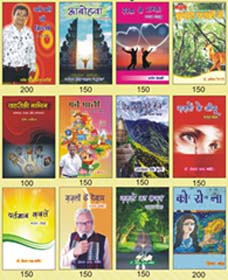Previous Next2021 में प्रकाशित कुछ नयी पुस्तकें

## All Arrivals

Rs. 200 Rs. 198

Rs. 250 Rs. 225

Rs. 500 Rs. 375

Rs. 250 Rs. 225

Rs. 250 Rs. 225

Rs. 250 Rs. 225

Rs. 180 Rs. 162

Rs. 200 Rs. 198

Rs. 250 Rs. 225

Rs. 150 Rs. 135

Rs. 250 Rs. 225

Rs. 400 Rs. 380

Rs. 250 Rs. 225

Rs. 250 Rs. 225

Rs. 150 Rs. 135

##### Shakh - E - Ghazal

Rs. 275 Rs. 247.5

Rs. 200 Rs. 180

Rs. 90 Rs. 81

Rs. 110 Rs. 99